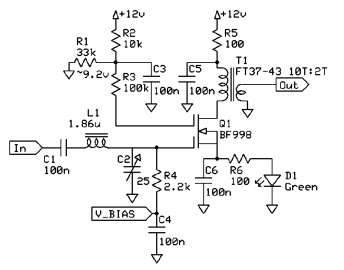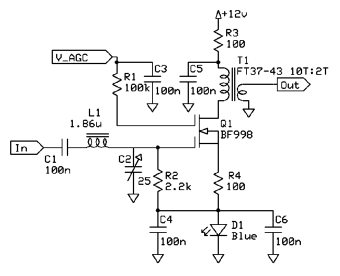# Dual Gate MOSFET Investigations – Gain and AGC

Having determined some basic characteristics of the biasing of the BF998 dual gate MOSFET in a previous experiment, it was now time to look into the gain and AGC performance of the amplifier. A few changes were made to the original circuit to turn it into a proper RF amplifier.

#### Test Equipment

• Power Meter: M3 Electronix FPM-1
• Voltmeter: Fluke 8840A
• Signal Generator: Tektronix SG503

#### Initial Test Conditions

The gate 1 voltage (VBIAS) was initially biased to 3.16 V, a level that was previously determined to give about 10 mA of drain current when gate 2 is biased to 9.2 V. The input signal was set to a frequency of approximately 28.1 MHz, to give an idea of the amplifier performance in the upper HF bands. The output power of the signal generator was set to -30.0 dBm into a 50 Ω resistive load. This gave me enough power to make a good measurement with the FPM-1 while avoiding the problem of gain compression. All gain measurements are based off of this amplifier input power (in other words, the amplifier gain described in this report is the transducer gain).

#### The Circuit

The DC biasing of the circuit is virtually identical to the final configuration determined in the first experiment. However, there have been some changes in regard to the input and output circuitry. First of all, the gate 1 bias is now fed through a 2.2 kΩ resistor which is bypassed to AC ground with a 100 nF capacitor. This sets the input impedance to 2.2 kΩ. Values around 2 kΩ seem to be fairly common in the literature, apparently because of the noise figure benefits. I would like to investigate this further in a later experiment, but for now we’ll go with the wisdom of others. A typical L-network was placed on the input to transform the 50 Ω amplifier input impedance to the 2.2 kΩ impedance that gate 1 wants to see. The drain inductor was replaced with a 10:2 ratio transformer to give the drain a load of 1.25 kΩ to work into when a 50 Ω load is placed on the amplifier output. Again, this is another area where I decided to go with the wisdom of others. This drain load values seems reasonable based on other FET amplifiers I’ve used, but it might also be an area worth investigating later.#### Results

Under the initial conditions described above, I measured an output power of -6.1 dBm, which indicates a transducer gain of 23.9 dB. This seems like a reasonable and believeable amount of gain from a single amplifier given the biasing levels established. I decided to vary VBIAS a bit to determine the point of maximum gain for the amplifier. At a gate 1 voltage of 3.43 V, I measured -5.9 dBm of output power, or a gain of 24.1 dB. There is a slight amount of difference between the two voltages, but not enough to be significant. It seems that the initial estimate worked fairly well.

#### AGC Characteristics

Next, the circuit was modified slightly to examine the AGC characteristics of the BF998. Both the source and gate 1 were biased to approximately 3 V using a blue LED. This biasing method is very convenient, simple, and stable, even if it may not bias gate 1 to its ideal point. This reduced the drain current to 6.6 mA, which would mean a slightly lower maximum gain, but also would be a more power-efficient way to run the amp. I could have used two red or green diodes in series, or a string of small-signal diodes as seen in the Hybrid Cascode amplifier, but the blue LED uses the least parts (and is pretty to boot). The fixed voltage divider bias was removed from gate 2, and in its place a variable AGC voltage (VAGC) was applied. The same -30.0 dBm input power was used, and the output power was measured at different settings of VAGC.#### Results

As you can see in the table and chart below, there is a large AGC voltage range with very little gain variation, then a sharp knee where there is a steep slope of gain reduction. The knee occurs at an AGC voltage of about 3 V. Between 2 V and 3 V is the largest gain variation (about 40 dB). This AGC response curve actually appears to agree fairly well with the curve published in the Philips RF Manual 3rd Edition Appendix for the BF998. The AGC range of approximately 50 dB also seems in line with the data published by NXP. It does look plausible that two of these amplifiers cascaded together could provide nearly 100 dB of gain reduction (another experiment idea for later).

VAGC POUT GT
2 V -55.7 dBm -25.7 dB
2.25 V -52.8 dBm -22.8 dB
2.5 V -37.2 dBm -7.2 dB
2.75 V -23.3 dBm 6.7 dB
3 V -16.0 dBm 14.0 dB
4 V -12.6 dBm 17.4 dB
5 V -11.2 dBm 18.8 dB
6 V -10.4 dBm 19.6 dB
7 V -9.9 dBm 20.1 dB
8 V -9.5 dBm 20.5 dB
9 V -9.1 dBm 20.9 dB
10 V -9.0 dBm 21.0 dBNext, I intend to build a return loss bridge (finally!) and get some measurements on this amplifier. I also need to look into what it will take to measure noise figure, and get started on that test rig as well.

## 6 thoughts on “Dual Gate MOSFET Investigations – Gain and AGC”

1.kba

Hello OM

Just read the AGC characteristics with great interest…
One thing I became curious was the very odd AGC/gain constant
which varies so much as the gain decreases…
Did you happen to measure the fet Id current / gain dependency ? I have IF amplifier under construction and have plan to base the signal strength indication to fet Id current value which I believe is more linear in relation to gain reduction.
This takes some time to verify as measurement waits the whole
IF strip & AGC to be finished…

br Kari B
Oh6io

2.NT7S

Hello Kari,

I did not make the variable drain current measurements that you have described, but that would make for a great follow up experiment. The intriguing thing about dual-gate MOSFETs is the different ways that you can bias them. If you get around to that experiment before I do, please let me know what you find.

73,
Jason NT7S

3.Volker Kible

Hello Jason,

I’m trying to reproduce your measuerements with LTSpice, but it doesn’t really work, my amplifier doesn’t amplify. Maybe there’s something wrong with the model i’m using, or my circuit is not correct. I hope you have some experience with Spice and can help me. I’m new to Spice…

Thanx & Regards!
Volker

This is the model i’m using (i changed the original model of NXP [http://www.nxp.com/models/spicespar/fet/BF998.html] a bit to get it running in LTSpice IV, maybe i destroyed it):
* BF998 SPICE MODEL OCTOBER 1993 PHILIPS SEMICONDUCTORS
* ENVELOPE SOT143 (R)
* 1.: SOURCE; 2.: DRAIN; 3.: GATE 2; 4.: GATE 1;
.SUBCKT BF998 1 2 3 4
L10 1 10 0.12N
L20 2 20 0.12N
L30 3 30 0.12N
L40 4 40 0.12N
L11 10 11 1.20N
L21 20 21 1.20N
L31 30 31 1.20N
L41 40 41 1.20N
C13 10 30 0.085P
C14 10 40 0.085P
C23 20 30 0.085P
C24 20 40 0.005P
D11 42 11 ZENER
D12 42 41 ZENER
D21 32 11 ZENER
D22 32 31 ZENER
RS 10 12 100
MOS1 61 41 11 12 GATE1
MOS2 21 31 61 12 GATE2

.MODEL ZENER D(BV=10 CJO=1.2E-12 RS=10)

.MODEL GATE1
+ NMOS(W=1.1E-6 L=1150E-6 LEVEL=3 UO=600 VTO=-0.250 NFS=300E9 TOX=42E-9
+ NSUB=3E15 VMAX=140E3 RS=2.0 RD=2.0 XJ=200E-9 THETA=0.11
+ ETA=0.06 KAPPA=2 LD=0.1E-6
+ CGSO=0.3E-9 CGDO=0.3E-9 CBD=0.5E-12 CBS=0.5E-12)

.MODEL GATE2
+ NMOS(W=2.0E-6 L=1150E-6 LEVEL=3 UO=600 VTO=-0.250 NFS=300E9 TOX=42E-9
+ NSUB=3E15 VMAX=100E3 RS=2.0 RD=2.0 XJ=200E-9 THETA=0.11
+ ETA=0.06 KAPPA=2 LD=0.1E-6
+ CGSO=0.3E-9 CGDO=0.3E-9 CBD=0.5E-12 CBS=0.5E-12)

.ENDS BF998

4.Edwin Richter DC9OE

Hi Volker, not sure you solved your problem alrrady… 🙂 I spent the evening doing exactly the same simulation and when finished I looked at what conclusions other people have drawn ( my simulations came to the same result more or less to what is described abobve ).

In your model above you need to take away the L’s and the C’s.

This is described in a very good tutorial at

http://elektronikschule.de/~krausg/

( available in english as well )

Kind regards, Edi DC9OE

5. […] should have output impedance 200ohm? Then after the IF filter I have this dual gate MOSFET with AGC http://nt7s.com/2008/07/dual-gate-mo…-gain-and-agc/ as a IF amplifier. And I am confused with if I need impedance matching circuit between IF filter […]

6.ranjini rao

Hello
I dont know to write spice model(large signal model) for below S-parameters. Please send me the model.
* BF998 SPICE MODEL OCTOBER 1993 PHILIPS SEMICONDUCTORS
* ENVELOPE SOT143 (R)
* 1.: SOURCE; 2.: DRAIN; 3.: GATE 2; 4.: GATE 1;
.SUBCKT BF998 1 2 3 4
L10 1 10 0.12N
L20 2 20 0.12N
L30 3 30 0.12N
L40 4 40 0.12N
L11 10 11 1.20N
L21 20 21 1.20N
L31 30 31 1.20N
L41 40 41 1.20N
C13 10 30 0.085P
C14 10 40 0.085P
C23 20 30 0.085P
C24 20 40 0.005P
D11 42 11 ZENER
D12 42 41 ZENER
D21 32 11 ZENER
D22 32 31 ZENER
RS 10 12 100
MOS1 61 41 11 12 GATE1
MOS2 21 31 61 12 GATE2
.MODEL ZENER D(BV=10 CJO=1.2E-12 RS=10)
.MODEL GATE1
+ NMOS(W=1.1E-6 L=1150E-6 LEVEL=3 UO=600 VTO=-0.250 NFS=300E9 TOX=42E-9
+ NSUB=3E15 VMAX=140E3 RS=2.0 RD=2.0 XJ=200E-9 THETA=0.11
+ ETA=0.06 KAPPA=2 LD=0.1E-6
+ CGSO=0.3E-9 CGDO=0.3E-9 CBD=0.5E-12 CBS=0.5E-12)
.MODEL GATE2
+ NMOS(W=2.0E-6 L=1150E-6 LEVEL=3 UO=600 VTO=-0.250 NFS=300E9 TOX=42E-9
+ NSUB=3E15 VMAX=100E3 RS=2.0 RD=2.0 XJ=200E-9 THETA=0.11
+ ETA=0.06 KAPPA=2 LD=0.1E-6
+ CGSO=0.3E-9 CGDO=0.3E-9 CBD=0.5E-12 CBS=0.5E-12)
.ENDS BF998